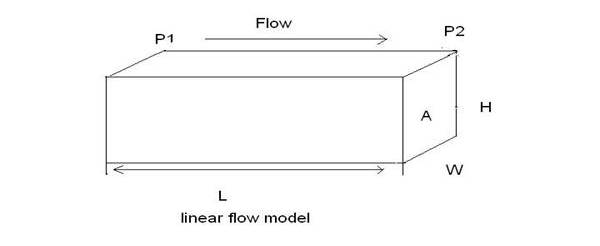# Permeability Assignment Help

## Permeability

Permeability is a property of the porous medium that measures the capacity and ability of the formation to transmit fluids. The rock permeability, k, is a very important rock property because it controls the directional movement and the flow rate of the reservoir fluids in the formation.This rock characterization was first defined mathematically by Henry Darcy in 1856. In fact, the equation that defines permeability in terms of measurable quantities is called Darcy's Law. Darcy developed a fluid flow equation that has since become one of the standard mathematical tools of the petroleum engineer. If a horizontal linear flow of an incompressible fluid is established through a core sample of length L and a cross-section of area A, then the governing fluid flow equation is defined as

K dP

V = -- ---- ----

µ dL

Where n = apparent fluid flowing velocity, cm/sec

K = proportionality constant, or permeability, Darcys

m = viscosity of the flowing fluid, cp

Dp/dL = pressure drop per unit length, atm/cm

The velocity, n, in above equation is not the actual velocity of the flowing fluid but is the apparent velocity determined by dividing the flow rate by the cross-sectional area across which fluid is flowing.

Substituting the relationship, q/A, in place of n in above equation and solving for q results in…

KA dP

Q = --- ------……..1

µ dL

Where Q = flow rate through the porous medium, cm3/sec

A = cross-sectional area across which flow occurs, cm2

## Permeability Assignment Help By Online Tutoring and Guided Sessions at AssignmentHelp.Net

With a flow rate of one cubic centimeter per second across a cross-sectional area of one square centimeter with a fluid of one centipoises viscosity and a pressure gradient at one atmosphere per centimeter of length, it is obvious that k is unity. For the units described above, k has been arbitrarily assigned a unit called Darcy in honor of the man responsible for the development of the theory of flow through porous media. Thus, when all other parts of above equation have values of unity, k has a value of one Darcy.

One Darcy is a relatively high permeability as the permeability of most reservoir are less than one Darcy. In order to avoid the use of fractions in describing perm abilities, the term millidarcy is used. As the term indicates, one millidarcy, i.e., 1 md, is equal to one-thousandth of

One Darcy or,

1 Darcy = 1000 md

The negative sign in Equation 1 is necessary as the pressure increases in one direction while the length increases in the opposite direction. Equation 1 can be integrated when the geometry of the system through which fluid flows is known. For the simple linear system shown in Figure 4-19, the integration is performed as follows:

qL = -- kA / µ (p2-p1)

It should be pointed out that the volumetric flow rate, q, is constant for liquids because the density does not change significantly with pressure. Since p1 is greater than p2, the pressure terms can be rearranged, which will eliminate the negative term in the equation. The resulting equation is….

KA (P1—P2)

Q = ------………2Fig 1. Linear flow model

Equation 2 is the conventional linear flow equation used in fluid flow calculations. Standard laboratory analysis procedures will generally provide reliable data on permeability of core samples. If the rock is not homogeneous, the whole core analysis technique will probably yield more accurate results than the analysis of core plugs (small pieces cut from the core). Procedures that have been used for improving the accuracy of the permeability determination include cutting the core with an oil-base mud, employing a pressure- core barrel, and conducting the permeability tests with reservoir oil. Permeability is reduced by overburden pressure, and this factor should be considered in estimating permeability of the reservoir rock in deep wells because permeability is an isotropic property of porous rock in some defined regions of the system, that is, it is directional. Routine core analysis is generally concerned with plug samples drilled parallel to bedding planes and, hence, parallel to direction of flow in the reservoir. These yield horizontal permeabilities (kh). There are several factors that must be considered as possible sources of error in determining reservoir permeability.

These factors are:

1. Core sample may not be representative of the reservoir rock because of reservoir heterogeneity.

2. Core recovery may be incomplete.

3. Permeability of the core may be altered when it is cut, or when it is cleaned and dried in preparation for analysis. This problem is likely to occur when the rock contains reactive clays.

4. Sampling process may be biased. There is a temptation to select the best parts of the core for analysis.